# Turtles 2

A box turtle hibernates in the sand at 11 5/8. A spotted turtle hibernates at 11 16/25 feet. Which turtle is deeper? Write answer as number 1 or 2.

x =  2

### Step-by-step explanation:Did you find an error or inaccuracy? Feel free to write us. Thank you!

Showing 1 comment:Matematik
For calculation fraction in feet please checkout our fraction calculator:

https://www.hackmath.net/en/calculator/fraction?input=13%27+5%27%27+1%2F2+%2B+16%27+4%27%27+3%2F8%3D&submit=Calculate

It can handle more complex expression with mixing ft and inches like 13' 5'' 1/2 + 16' 4'' 3/8=Tips to related online calculators
Need help to calculate sum, simplify or multiply fractions? Try our fraction calculator.
Do you want to convert length units?

## Related math problems and questions:

• Linear combination of complex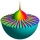If z1=5+3i and z2=4-2i, write the following in the form a+bi a) 4z1+6z2 b) z1*z2
• Simplify 3Simplify mixed numerals expression: 8 1/4- 3 2/5 - (2 1/3 - 1/4) Show your solution.
• Sum of fractionsWhat is the sum of 2/3+3/5?
• Mixed numbersRewrite mixed numbers, so the fractions have the same denominator: 5 1/5 - 2 2/3The heights of five starters on redwood high’s basketball team are 5’11”, 6’3”, 6’6”, 6’2” and 6’. The average of height of these players is?
• BoatsThree-quarters of boats are white, 1/7 are blue and 9 are red. How many boats do we have?
• A turtle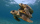A turtle is 20 5/6 inches below the surface of a pond. It dives to a depth of 32 1/4 inches. What is the change in the turtle’s position? Write your answer as a mixed number.
• Evaluate 5Evaluate expression x2−7x+12x−4 when x=−1
• LCD two fractionsWhat is the least common denominator of 11/15 and 12/19?
• Fraction + eqSolve following simple equation with fractions: -5/6(8+5b) = 75 + 5/3b
• Subtracting complex in polar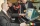Given w =√2(cosine (p/4) + i sine (pi/4) ) and z = 2 (cosine (pi/2) + i sine (pi/2) ), what is w - z expressed in polar form?
• Statistical XY file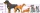Year; money spent on advertising; profit (three values each row) 2008 2 12 2009 5 20 2010 7 25 2011 11 26 2012 15 40 1. draw a scatter diagram depicting the data. 2. calculate the Pearson's correlation coefficient. 3. determine the linear regression equat
• Prime factorsWrite 98 as a product of prime factors
• Emily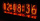Emily had 20 minutes to do a three-problem quiz. She spent 11 3/4 minutes on question A and 5 1/2 minutes on question B. How much time did she have left for question C?
• ComplaintsThe table is given: days complaints 0-4 2 5-9 4 10-14 8 15-19 6 20-24 4 25-29 3 30-34 3 1.1 What percentage of complaints were resolved within 2weeks? 1.2 calculate the mean number of days to resolve these complaints. 1.3 calculate the modal number of day
• Eq2 2Solve following equation with quadratic members and rational function: (x2+1)/(x-4) + (x2-1)/(x+3) = 23
• Box and whisker plotConstruct a box and whisker plot for the given data. 56, 32, 54, 32, 23, 67, 23, 45, 12, 32, 34, 24, 36, 47, 19, 43